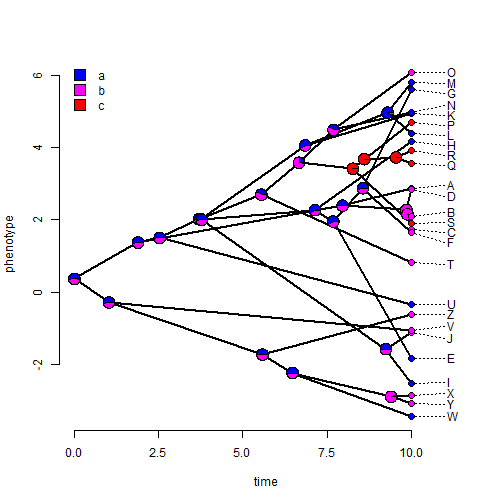## Saturday, February 6, 2016

### Update to `phenogram` to be used with `nodelabels`, `tiplabels` (plus a small bug fix)

I just added the feature that the traitgram plotting function in phytools, `phenogram`, will now also assign the environmental variable `"last_plot.phylo"` which will allow users to add things like tip, node, and edge labels to a plotted traitgram created using this function.

Details of this update can be viewed on the phytools GitHub page here, along with a fix for a bug in `phenogram(...,spread.labels=TRUE)` when the labels are non-overlapping to begin with.

Here's a demo in which I will overlay the observed & estimated ancestral values for a discrete character onto a plotted traitgram:

``````library(phytools)
packageVersion("phytools")
``````
``````##  '0.5.16'
``````
``````tree
``````
``````##
## Phylogenetic tree with 26 tips and 25 internal nodes.
##
## Tip labels:
##  A, B, C, D, E, F, ...
##
## Rooted; includes branch lengths.
``````
``````x
``````
``````##       A       B       C       D       E       F       G       H       I
##  2.8600  2.1058  1.7397  2.8509 -1.8374  1.6606  5.6038  4.1693 -2.5189
##       J       K       L       M       N       O       P       Q       R
## -1.1314  4.9547  4.3946  5.8012  4.9661  6.0724  4.6994  3.5680  3.9083
##       S       T       U       V       W       X       Y       Z
##  1.8861  0.8216 -0.3356 -1.0667 -3.4524 -2.8598 -3.0773 -0.6128
``````
``````y
``````
``````## A B C D E F G H I J K L M N O P Q R S T U V W X Y Z
## a b b b a b a a a b a a a a b c c c c b a b a b b b
## Levels: a b c
``````
``````phenogram(tree,x)
obj<-rerootingMethod(tree,y,model="SYM")
cols<-setNames(c("blue","magenta","red"),
colnames(obj\$marginal.anc))
nodelabels(pie=obj\$marginal.anc,piecol=cols,cex=0.7)
tiplabels(pie=to.matrix(y,seq=colnames(obj\$marginal.anc)),
piecol=cols,cex=0.4)
``````This version of phytools can be installed as follows:

``````library(devtools)
install_github("liamrevell/phytools")
``````

The data for this example were simulated using the following code:

``````tree<-pbtree(n=26,tip.label=LETTERS,scale=10)
x<-round(fastBM(tree),4)
Q<-matrix(c(-0.25,0.25,0,
0.25,-0.5,0.25,
0,0.25,-0.25),3,3)
rownames(Q)<-colnames(Q)<-letters[1:3]
y<-sim.history(tree,Q)\$states
y<-setNames(as.factor(y),names(y))
``````

1.This comment has been removed by the author.

2.Hi Liam,

Really neat function and I'm excited to use it! However, I'm encountering a couple (possibly interrelated) glitches. I was hoping you could illuminate my as to whether they are due to my ignorance or to there really being bugs in the function.

1. Some branches don't go all the way to the end of the x-axis (i.e., some tips are in the middle of the plot, as if the tree wasn't ultrametric).

2. Tip labels simply aren't plotted

The tree is of type simmap and when I plot it with plot.Simmap(), everything is normal.

Any hints?

Thanks!

Note: due to the very large amount of spam, all comments are now automatically submitted for moderation.